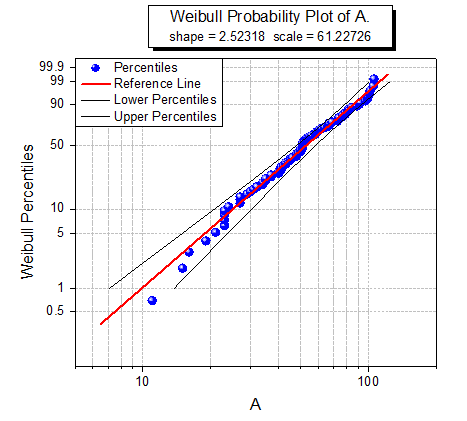Skip Navigation Links.

Weibull Probability PlotLearn how to create this graph here.

The probability plot is a graph with observed cumulative percentage on the X axis and expected cumulative percentage on the Y axis. The Weibull probability plot is used to test whether or not a dataset follows the Weibull distribution. And its X scale type and Y scale type are Log10 and Double Log Reciprocal respectively. If all the scatter points are close to the reference line, we can say that the dataset follows the Weibull distribution.

Note that Origin supports to output the confidence band in the Weibull Probability plot by selecting the Confidence Band check box since Origin 2016.

This graph is a typical Weibull probability plot in Origin.